# Deep Learning — Week 2

Last week, we touched upon what a neural network actually does and introduced Deep Learning in brief. If you missed last week’s article, you can find it here.

This week, we’ll dive right in and start off with the core concepts of Deep Learning in pure mathematical detail. After reading this article, you’ll be able to understand:

1. Structures of different types of neurons
2. The necessity of objective functions, loss functions and activation functions
3. The learning algorithm employed in order to help the network draw relations between the outputs and the inputs
4. Why Gradient Descent is such a big deal for optimization
5. How a neural network rectifies itself to minimize error/ loss

If you want to follow along with the video lectures this series is inspired from, you can tune in to this playlist on YouTube.

# How a simple Neural Network works:

Neural Networks are a condensed, ripped-off version of the way our brain functions. Our nervous system is made up of millions of neurons connected to each other sequentially. The brain makes decisions based on which neurons fire and by how much. For example, when you’re watching The Office and come across a joke, what do you think happens in that skull of yours?

• There is a massively parallel interconnected network of neurons.
• The sense organs relay information (input) to the lowest layer of neurons.
• Some of these neurons may fire and may relay the information to the next few layers of neurons.
• Each neuron performs a certain role or responds to a certain stimulus.
• This massively parallel network also ensures division of labour.
• This process continues till the topmost layer of neurons in the brain, which will fire only when the firing neurons in the penultimate layer are more than a certain threshold level (here, it’s 2 out of 3 neurons).

It’s as simple as that.
Your neural network extracts meaningful information from the given inputs, relays it on to make draw further insights and features and provides the result as the output.

Artificial neural networks work with the exact same principle. Deep Learning focusses on using input data to figure out patterns within them, or determine mathematical relations between the inputs and the outputs which can be used to further extrapolate and predict trends based on previous inputs.

What we saw just now is an overly simplified version of how the brain works, but it suffices for our discussion on Neural Nets. Before understanding how a complete network functions, it’s essential to look at how individual neurons work and how they can be modified to suit different needs.

In 1943, the simplest computational model of a neuron (McCulloch-Pitts Neuron) was introduced.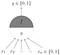‘g’ aggregates the inputs and ‘f’ takes a decision based on this aggregation.

The inputs can be excitatory (1) or inhibitory (0).

In the traditional model, y = 0 if the sum of all inputs is lesser than a given threshold.

Based on the above criteria, we can replicate boolean functions using a single neuron: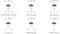*circle at the end indicates inhibitory input: if any inhibitory input is 1 then output will be 0.

As you probably noticed, McCulloch-Pitts Neuron doesn’t come without its own set of limitations.

• What about non-boolean (real valued) functions?
• Do we need to set the threshold by hand?
• What if we want to assign more importance to some inputs?

To counter these short-comings, the perceptron was introduced in 1958. The main differences are:

• Inputs are no longer restricted to boolean values
• Numerical weights introduced for each input
• There exists a mechanism (algorithm) to learn these weights automatically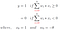‘Theta’ is the threshold, ‘w’ are the weights while ‘x’ are individual inputs.

Hence, a perceptron can assign more weightage to certain inputs and take decisions based on how the collective sum fares against the threshold value.

However, the threshold logic of a perceptron is a bit too harsh.
Consider an example: you’re building a single-neuron system which will decide if a movie is worth going to. You set the threshold to a rating of 0.5 out of 1.

According to this system, the switch from “no, this movie is trash” to “yes, this movie deserves an Oscar” is rather too immediate.

Moreover, this system would return y = 0 (do not watch) if the ratings were 0.49, which is rather strange as it is close to 0.5 (which happens to be my threshold).

What we need, is a system response that has a smoother decision function.

This calls for a change in the function employed by neurons. Introducing a sigmoid neuron can help with a gradual function suitable for real-world applications.

Sigmoid function is a family of functions, some of which are:

• tanh function
• Logistic function
• Softmax function

Furthermore, the output is not binary, but is a real-valued decimal which can be interpreted as probability.

An example of the sigmoid function is the logistic function (in graph):

Sigmoid functions are also smooth, continuous and differentiable at all points.

Neurons are used to derive insights into certain patterns posed by input data to obtain mathematical functions that best fit the input data points with minimal error.

A McCulloch-Pitts neuron can generate a linear function in n-dimensions (a line, or a plane or a hyperplane). It only holds good for linearly separable functions (functions which return y = 1 above the line/plane and return y = 0 below the line/plane).

However, it is not possible to generate a non-linear function which can accurately classify the red points and blue points separately (in the adjacent figures) using a single neuron.

To generate non-linear functions that can approximately traverse between points to classify most of them accurately, you’ll have to use a network of neurons interconnected layer-wise. Each layer finds different patterns and transmits forward, where they become more profound.

You can try working on different functions in the Neural Network Playground from TensorFlow. Its fun, its foolproof, and you don’t need to be a nerd to be able to use it.

So based on individual neuron behaviour, we present a fully working artificial feed-forward neural network!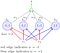An artificial neural network. Notice how each input is connected to every neuron.

Terminology:

1. This network contains 3 layers.
2. The layer containing the inputs is called the input layer.
3. The middle layer containing the 4 perceptrons is called the hidden layer.
4. The final layer containing the output neuron is called the output layer.
5. The outputs of the 4 perceptrons in the hidden layer are denoted by h1, h2, h3 and h4.
6. The red and blue edges are called layer 1 weights.
7. w1, w2, w3 and w4 are called layer 2 weights.

In order to enable the neural network replicate the desired function with minimal error, we need to employ some techniques which will ensure that the standard output function (sigmoid function/ perceptron output summation) is moving towards the function we’re looking for. One way to gauge the accuracy of the output with respect to the function is by using a loss function.

Loss functions are metrics that help in keeping track of how distant the outputs of the neural network are from the desired function values. One example of loss functions is the famous squared error loss: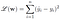‘y-hat’ is the output of the neural network. ‘y’ is the expected output from the desired function.

We know, from a single neuron we have: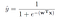‘w’ stands for the weights employed in the network. Remember, it is a matrix whose elements are individual weights. ‘x’ is a vector containing the inputs.

With a combination of these neurons, we will end up getting an arbitrary output in the first iteration, i.e. when we provide inputs and random weights in the beginning. We need the neural network to learn how the weights ‘w’ can be altered in order to bring the output function (y-hat) as close to the desired function as possible. (The closeness to the desired function is determined by the value of the loss function).

So we’ve got ourselves an optimization problem: minimize the loss function.

In order to minimize the loss function, we employ a matrix calculus based optimization technique called Gradient Descent.

You’ve previously seen that the neurons accumulate the inputs in the form (Wx + b) where ‘W’ and ‘b’ are the corresponding weight and bias respectively. Gradient Descent is a method to determine the required weights and biases by moving along the path opposite to the gradient so as to reach the global minima of the error surface.

Hold up. Catch a breather. Here’s everything you read about a network of neurons till now, ultra condensed:

1. You need to achieve a certain function exhibited by the points in your dataset.
2. You have functions such as the sigmoid and perceptron sum in your inventory. Your network of neurons need to find which parameters in your sigmoid function can be tweaked, and by how much, in order to bring your function as close to the desired one as possible.
3. Your function will be deemed closely accurate to the desired function if the loss function is minimal (ideal cases, zero).
4. Random weights are fed to the neural network along with the input.
5. The output is obtained for different values of x (different inputs). Of course, the output is arbitrary and is nowhere close to the function you want. Consequently, the loss function value is high.
6. Here’s where things get interesting and confusing (mainly because you’re going to hear names of topics that’re covered below, but don’t bother for now). Your neural network performs backpropagation (where all the gradients are computed) and subsequently performs gradient descent to ensure that new weights are assigned in a manner which decreases the loss function.
7. The network repeats the above step repeatedly till the loss reaches the global minima.

Well done, you’re much more confused than you were before. But stick around, and you’ll find out what backpropagation and gradient descent actually do. Then you can come back here and summarize the whole thing for yourself ;)

For example, in a simple neural network with perceptrons, we know:

Therefore, for a 2-layered neural network, we can write the output expressions as:

Thus, in Gradient Descent, we aim to make changes to the ‘W’ matrix and the ‘b’ vector in order to bring our output function as close to the desired function as possible, iteration-wise, slowly.

Thus, under Gradient Descent in each iteration, ‘w’ and ‘b’ are changed as follows:

Of course, the above step is performed iteratively to compute gradients, tweak ‘w’ and ‘b’, find the outputs for each neuron and compare them against the desired function using the loss function metric. The whole task is computationally intensive and requires quite a robust PC configuration, especially when you’re working with many layers, or images (they use specific preprocessing techniques like convolution and pooling before actually being fed into the neural network, so we’ll take those up in the coming weeks).

Check out what the code looks like. You’ll understand the algorithm better.

Thus, in a feedforward neural network we’ve observed that a function can be closely replicated by helping it learn how to minimize the error. Also, each neuron has a pre-activation and an activation part.

Pre-activation is basically the weighted sum of all inputs, and is represented as ‘a’.

Activation function is what converts the weighted sum into a point on the curve of the desired function. For example, a sigmoid neuron with the logistic function as the activation function will perform as:

We’ll represent the activation function output f(x) as ‘h’ here on.
Some commonly used activation functions are the softmax, linear, tanh, relu, etc. They’re relatively easier to grasp (after all, they’re just mathematical formulae) and you can read up on effect of activation online.

## Backpropagation:

We saw in gradient descent that we need to compute the gradients of the loss function with respect to all parameters (weights and biases) in order to make suitable changes to them in the next iteration.

How can we possibly do that in a network with ‘L’ layers and ’n’ different inputs? More importantly, how do we intuitively hold the weights and biases responsible for the disparity of the loss function at each iteration?

Let us consider the case of a thin, but dense neural network as shown below.

In order to find the gradient of the loss function with respect to the first set of weights (W111), we employ the chain rule:

In short, we get a certain loss ‘L(theta)’ at the output and we try to figure out who’s responsible for that loss. So we proceed as follows: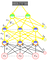Note that the yellow arrows just signify the direction of chain rule. The output is “talking” to each weight to find who’s responsible for the error.
1. The output layer talks to the two neurons below and tells them to take up responsibility.
2. These two neurons are only as good as the ones further connected to them below.
After all,
f(x) = activation(Wh + b)
3. The activation layer then communicates with the pre-activation layer, which further states that the problem lies with the activated outputs from the layer beneath.
4. This trend continues till the final layer of inputs, where we realize that all the responsibility lies with the parameters of the model, i.e. the weights and biases.

But instead of talking to them directly, it is easier to talk to them via the hidden layers and the output layers. And that is exactly what the chain rule allows us to do.

The above expression returns the gradient with respect to W111 alone. Similar expressions need to be computed for gradients with respect to all other weights and biases; a very computationally intensive task.

Let us look back and reflect on the algorithm for a feedforward neural network:

1. Initialize all weights and biases and perform forward propagation to set all pre-activation, activation functions and the output function.
Perform the below steps iteratively for a fixed number of epochs:
2. Initiate backpropagation:
- Compute the gradient w.r.t. the parameters
- Compute the gradient w.r.t. the activation functions
- Compute the gradient w.r.t. pre-activation layers below
3. Stack the obtained gradients along the rows of a matrix ‘theta’.
4. Use the obtained matrix to update the weights and biases using the gradient descent update rule.

5. As stated before, all the steps above will be iteratively performed.

This is a highly condensed and the most beginner friendly yet comprehensive explanation of backpropagation that can be provided. If you’re interested, you can knock yourself out with some YouTube explanations on Backpropagation, or check out Andrew Ng’s Deep Learning course on Coursera. Additionally, here’s the Deep Learning playlist again (if you missed the link above).

That’s week 2 for Deep Learning from FACE. Stay tuned on our Medium page and on Instagram, more content coming up next week. Until then, cheers and stay safe!

We are the departmental forum for Computer Science & Engineering, at Amrita School of Engineering , Bengaluru.

## More from FACE_ASEB

We are the departmental forum for Computer Science & Engineering, at Amrita School of Engineering , Bengaluru.